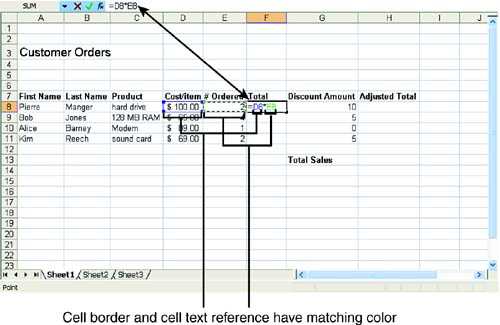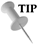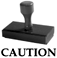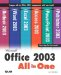# Entering Formulas

You can enter formulas in one of two ways: by typing the entire formula, including the cell addresses, or by typing the formula operators and selecting the cell references. Take a look at both ways.

To type a formula, perform the following steps:

1. Select the cell where you will place the formula.

2. Type an equal sign ( = ) into the cell to begin the formula.

3. Enter the appropriate cell references and operators for the formula. Figure 3.2 shows a simple multiplication formula. The formula also appears in the Formula bar as you type it. The cells that you specify in the formula are highlighted with a colored border.

##### Figure 3.2. The formula appears in the cell and in the Formula bar as you type it.4. Press Enter when you have finished the formula, and Excel calculates the result.Unwanted Formula If you start to enter a formula and then decide you don't want to use it, you can skip entering the formula by pressing Esc .

1. Click in the cell where you will place the formula.

2. Type the equal sign ( = ) to begin the formula.

3. Click the cell whose address you want to appear first in the formula. You can also click a cell in a different worksheet or workbook. The cell address appears in the cell and in the Formula bar.

4. Type a mathematical operator after the value to indicate the next operation you want to perform. The operator appears in the cell and in the Formula bar.

5. Continue clicking cells and typing operators until the formula is complete.

6. Press Enter to accept the formula and have Excel place its results into the cell.Error! If ERR appears in a cell, you probably made a mistake somewhere in the formula. Be sure you did not commit one of these common errors: dividing by zero, using a blank cell as a divisor, referring to a blank cell, deleting a cell used in a formula, or including a reference to the same cell in which the formula appears.Natural Language Formulas Excel also enables you to create what are called Natural Language formulas. You can refer to a cell by its column heading name and the corresponding row label. For example, if you had a column labeled Total and a column labeled Discount for each customer, you can write a formula such as =Smith TotalSmith Discount. You are referring to cells by the labels that you have placed in the worksheet rather than the actual cell addresses.Microsoft Office 2003 All-in-One
ISBN: B005HKSHB2
EAN: N/A
Year: 2002
Pages: 660
Authors: Joe Habraken

Similar book on Amazon